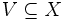# Locally operator

Jump to: navigation, search

## Definition

### Global "locally" operator

Let$p$ be a property of topological spaces. The property locally$p$ is defined as follows. A topological space$X$ is termed locally$p$ if it satisfies the following equivalent conditions:

1.$X$ has a basis such that all the members of the basis satisfy property$p$ when endowed with the subspace topology.
2. For any point$x \in X$ and any open subset$V \subseteq X$ such that$x \in V$, there exists an open subset$U$ of$X$ such that$x \in U \subseteq X$, and$U$ satisfies$p$ when given the subspace topology from$X$.

### "locally" at a point

Let$p$ be a property of topological spaces. A topological space$X$ is said to satisfy$p$ locally at a point$x \in X$ if the following holds: for any open subset$V \subseteq X$ such that$x \in V$, there exists an open subset$U$ of$X$ such that$x \in U \subseteq V$, and$U$ satisfies$p$ when given the subspace topology from$X$. The key difference with the global definition is that we are now imposing the condition relative to that particular point and not for all points.

## Facts

• If$p$ is a subspace-hereditary property of topological spaces, then being locally$p$ is equivalent to the condition that every point is contained in an open subset satisfying$p$. An example of this is the case that$p$ is the property of being a Hausdorff space, and locally$p$ is the property of being a locally Hausdorff space.
• If all spaces satisfying the property$p$ are self-based spaces, then being locally$p$ is equivalent to the condition that every point is contained an an open subset satisfying$p$. An example of this is the case that$p$ is the property of being a Euclidean space, and locally$p$ is the property of being a locally Euclidean space.
• If$p$ is a subspace-hereditary property of topological spaces, then$p$ implies locally$p$.
• There is a variant of the locally operator, called the strongly locally operator, and the prefix adjective locally is used for either of these. The strongly locally operator requires that for any neighbourhood of a point, there exists a smaller neighbourhood whose closure lies within the given neighbourhood, which has the required property. Strongly locally$p$ implies locally$p$.
• If$p$ is hereditary on open subsets, a regular space is locally$p$ iff it is strongly locally$p$.